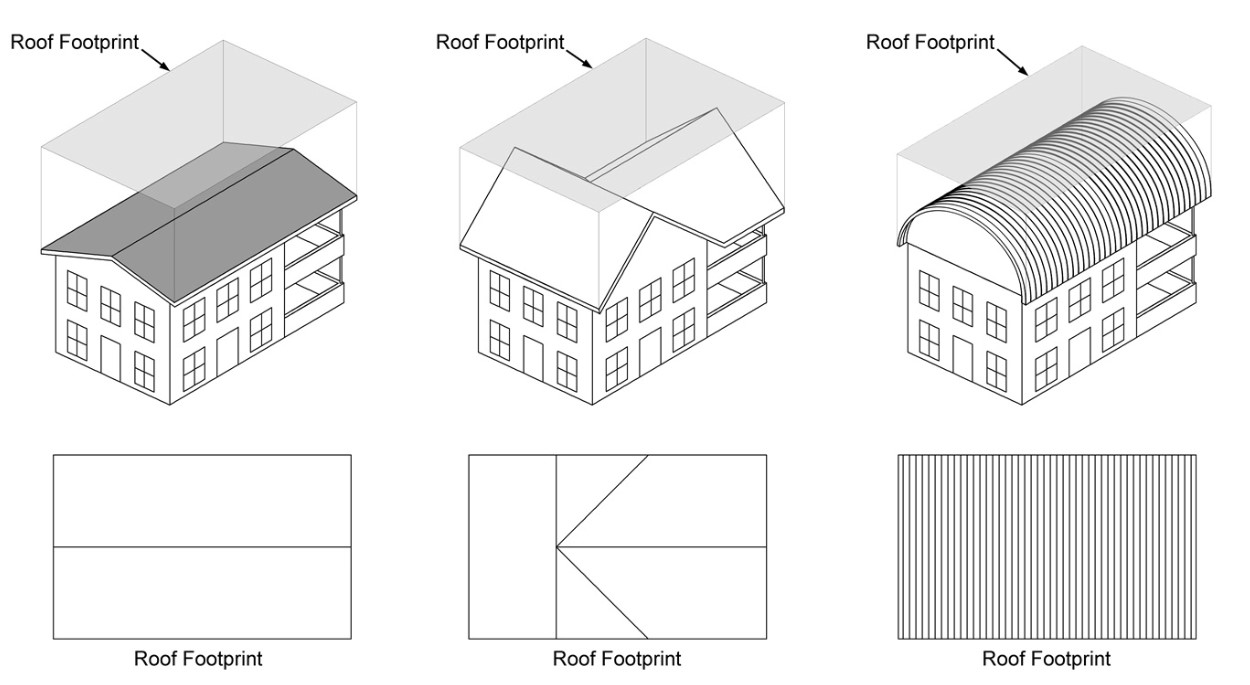# Rainwater Calculator

Look up your area's average annual rainfall totals here. For central Texas, the average annual rainfall is 32 inches.

### Don't Know Your Roof Area? Let's Calculate It.

To calculate the area of your roof, simply multiply the length (in feet) by width (in feet) for square or rectangular roofs. The resulting figure represents the surface area of your roof in square feet.

You don’t need to worry about the slope of your various roof plans. Most of the time, rain fall straight down and thus the angle or slope of your roof really doesn't matter in this calculation.  See the image below to help visualize the roof collection area.Source: Texas A&M Agrilife Extension

### Rainwater Collection Calculation Formulas and Equations

 Roof Area (ft2) X Precipitation Amount (in) X 0.623 = Amount Collected (gallons) Easy Formula to Remember: 1″ of Rain on 1,000 sf Roof will Yield 623 Gallons Roof Area (m2) X Precipitation Amount (mm) = Amount Collected (liters)